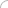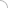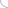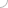## Chemistry quiz - online GK quiz

Home > GK > Chemistry

1) Which was the first metal that was used by man?

a) Gold.
b) Bronze.
c) Copper.
d) Iron.

2) Which among the following consists of the nucleus of an atom?

a) Electrons and neutrons.
b) Electrons and protons.
c) Protons and neutrons.
d) All of these.

3) Among the following which law states that the amount of gas dissolved in a liquid is proportional to its partial?

a) Henry’s law.
b) Raoult’s law.
c) Dalton’s law.
d) Gay Lussac’s law.

4) The nucleus of a hydrogen atom consists of:

a) 1 proton only.
b) 1 proton + 2 neutrons.
c) 1 neutron only.
d) 1 electron only.

5) Which among the following is the most abundant rare gas in the atmosphere?

a) He.
b) Ne.
c) Ar.
d) Xe.

6) What is the use of salt in the diet?

a) Make the taste of food better.
b) Produce in small amounts the hydrochloric acid required for the digestion of food.
c) Ease the process of cooking.
d) Increase the solubility of food particles in water.

ANSWER : Produce in small amounts the hydrochloric acid required for the digestion of food

7) The luster of a metal is due to:

a) Its high density.
b) Its high polishing.
c) Its chemical inertness.
d) Presence of free electrons.

ANSWER : Presence of free electrons

8) Which is the gas that is being used in the manufacture of vanaspati from vegetable oil?

a) Hydrogen.
b) Oxygen.
c) Nitrogen.
d) Carbon dioxide.

9) Among the following which is the most electropositive elements?

a) Na.
b) Ca.
c) K.
d) Cs.

10) Which is the gas that is used for artificial ripening of green fruit?

a) Carbon dioxide.
b) Acetylene.
c) Ethylene.
d) Ethane.

11) The high reactivity of fluorine is due to:

a) Its high electro negativity.
b) Small size of fluorine atom.
c) Availability of d-orbitals.
d) Strong F – F bond.

ANSWER : Its high electro negativity

12) The iron ore magnetite consists of:

a) Fe2O3
b) Fe3OH4

c) FeCO3
d) 3Fe2O3, 3H2O

13) Which among the following is most electronegative element?

a) Sodium.
b) Bromine.
c) Fluorine.
d) Oxygen.

14) Which is the most commonly used bleaching agent?

a) Alcohol.
b) Carbon dioxide.
c) Chlorine.
d) Sodium chlorine.

15) Which is the method that cannot be used to remove permanent hardness of water?

a) Distillation.
b) Boiling.

16) The number of moles of solute present in 1 kg of a solvent is called its………….

a) Molality.
b) Normality.
c) Formality.
d) Molarity.

17) Using which of the metallurgical process a metal is obtained in a fused state?

a) Roasting.
b) Froth flotation.
c) Calcinations.
d) Smelting.

18) Among the following the molecules of which gas have highest speed?

a) CH4 at 300K.
b) H2 at 73°C.
c) N2 at 1027°C.
d) O2 at 0°C.

19) The heat required to raise the temperature of body by 1 K is called:

a) Specific heat.
b) Thermal capacity.
c) Water equivalent.
d) None of these.

20) The octane number of zero is assigned to:

a) 2-methyl octane.
b) n-heptane.
c) iso-octane.
d) 3-methyl octane.General knowledge